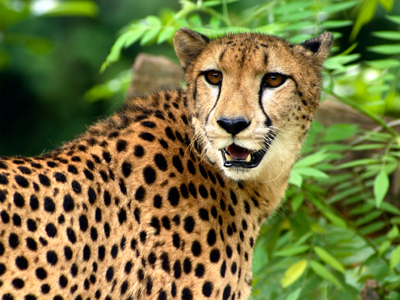A cheetah can run at 100km/h.

# Speed 3

This Science quiz is called 'Speed 3' and it has been written by teachers to help you if you are studying the subject at middle school. Playing educational quizzes is a fabulous way to learn if you are in the 6th, 7th or 8th grade - aged 11 to 14.

It costs only \$12.50 per month to play this quiz and over 3,500 others that help you with your school work. You can subscribe on the page at Join Us

Speed is how fast an object moves through time. Did you know that the average speed of a common snail is 0.001 m/s (meters per second), whereas the average orbital speed of planet Earth is 29,783 m/s?

Take your time and see how much you know about speed in our third Science quiz on the subject.

1.
If distance is measured in miles and speed in mph, time is measured in .......
seconds
minutes
hours
None of these
2.
How long would it take to travel 100km at a speed of 50km/h?
2 hours
60 minutes
5,000 seconds
5 hours
Time = distance/speed so 100km / 50km/h = 2 hours
3.
If distance is measured in meters and speed in m/s, time is measured in .......
seconds
minutes
hours
None of these
4.
How long would it take to run 800m at a speed of 8m/s?
80 seconds
100 seconds
1 hour
100 minutes
Time = distance/speed so 800m / 8m/s = 100s
5.
How long would it take to run 400m at a speed of 5m/s?
20 seconds
80 seconds
80 minutes
8 hours
Time = distance/speed so 400m / 5m/s = 80s
6.
How long would it take to travel 3km at a speed of 15m/s?
5 seconds
200 seconds
45 minutes
2 hours
3km = 3,000m so time = 3,000m / 15m/s = 200s
7.
A car travels at 70mph. How long will it take to travel 210 miles?
20 minutes
2.1 hours
3 hours
7 hours
Time = 210miles/70mph = 3 hours
8.
How long would it take to travel 300,000m at a speed of 60km/h?
0.5 hours
5 hours
5,000 minutes
5,000 hours
300,000m = 300km so time = 300km / 60km/h = 5 hours
9.
A cheetah can run at 100km/h. How long will it take to run 100 meters?
1 second
0.001 hours
1 minute
1 hour
100m = 0.1km so time = 0.1/100 = 0.001 hours, 1 hour = 3,600 seconds so 0.001h = 3.6 seconds!
10.
If distance is measured in kilometers and speed in km/h, time is measured in .......
seconds
minutes
hours
None of these
Author:  Sue Davison